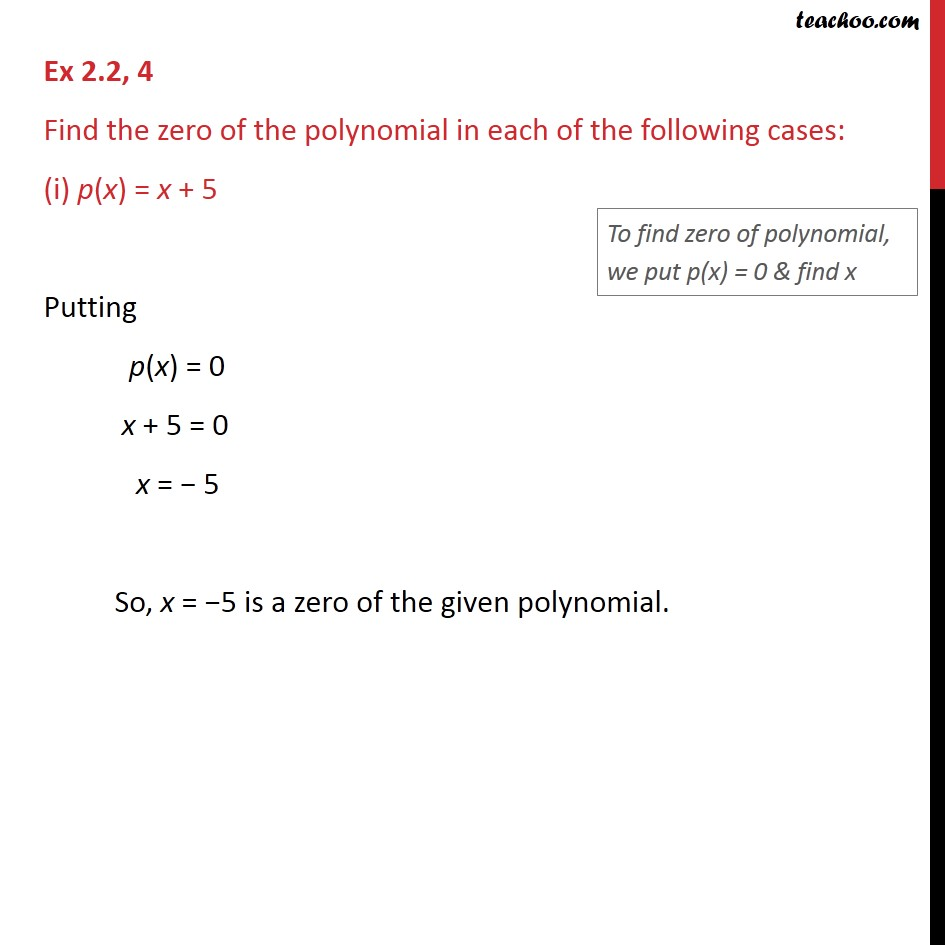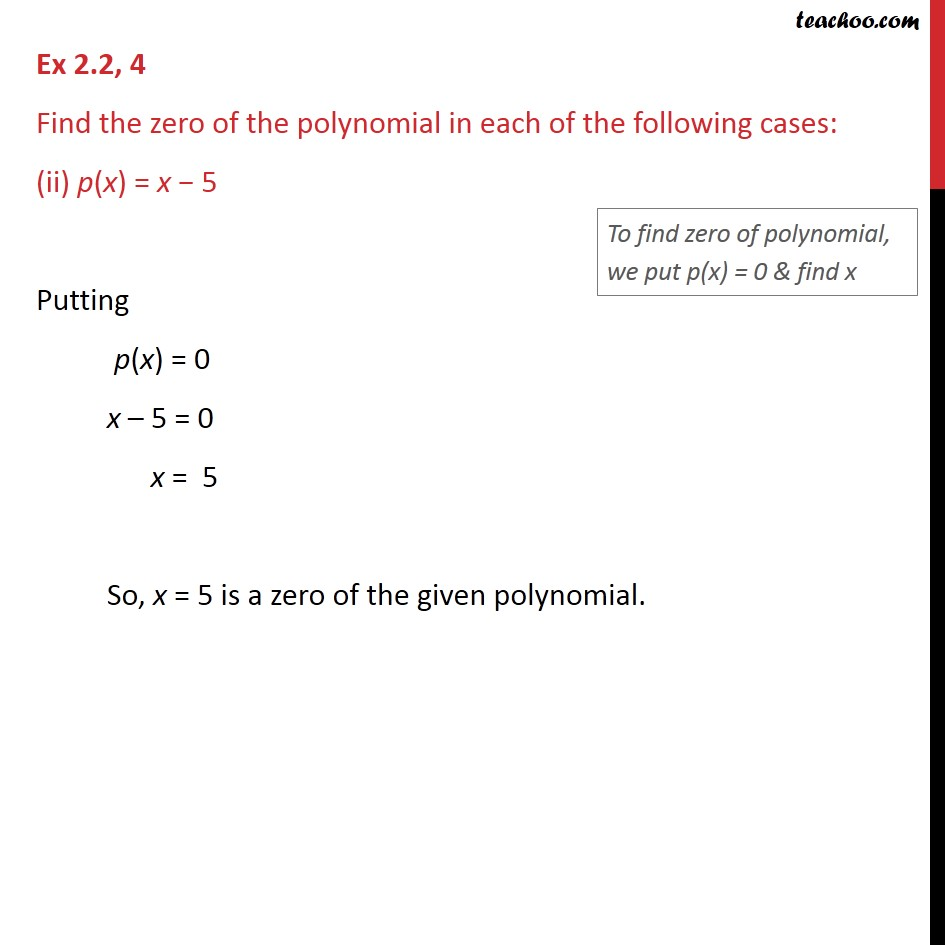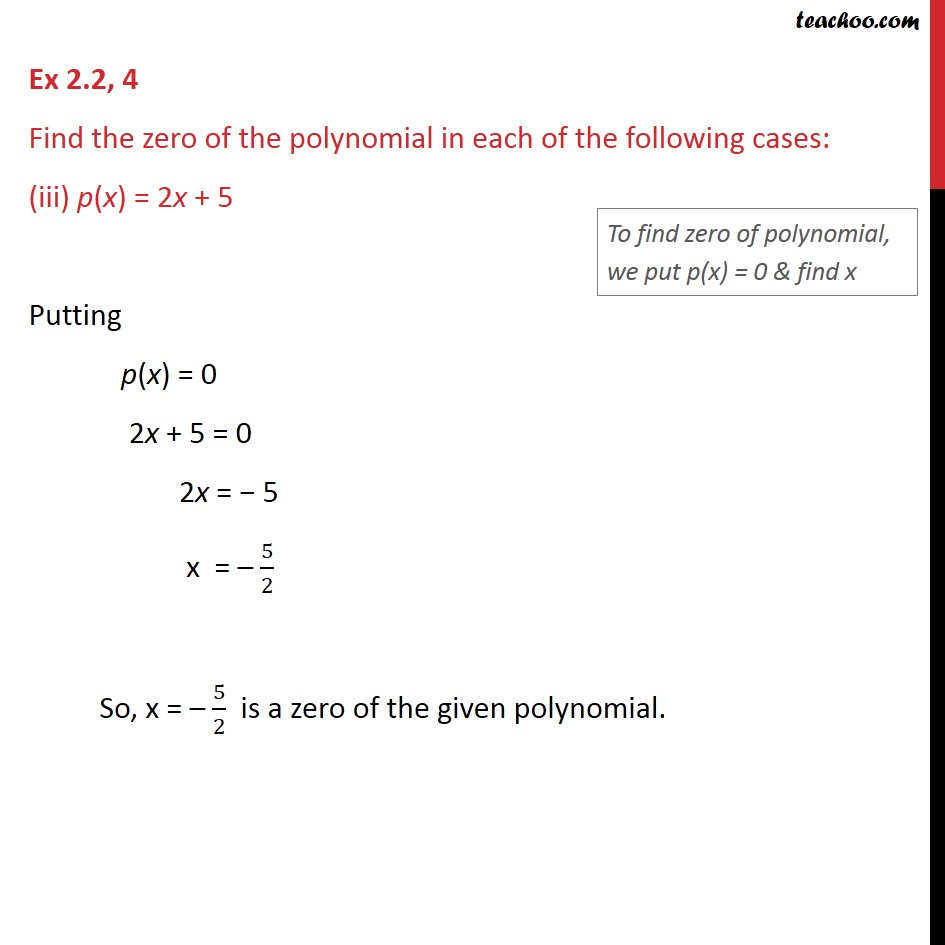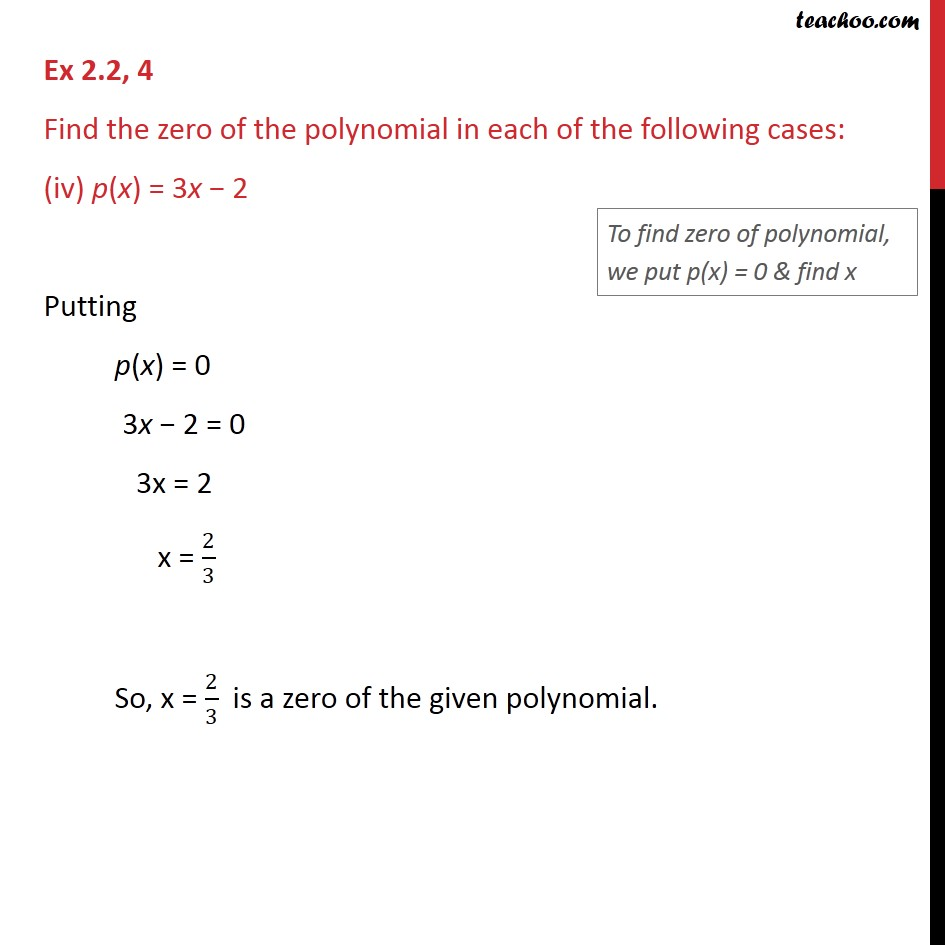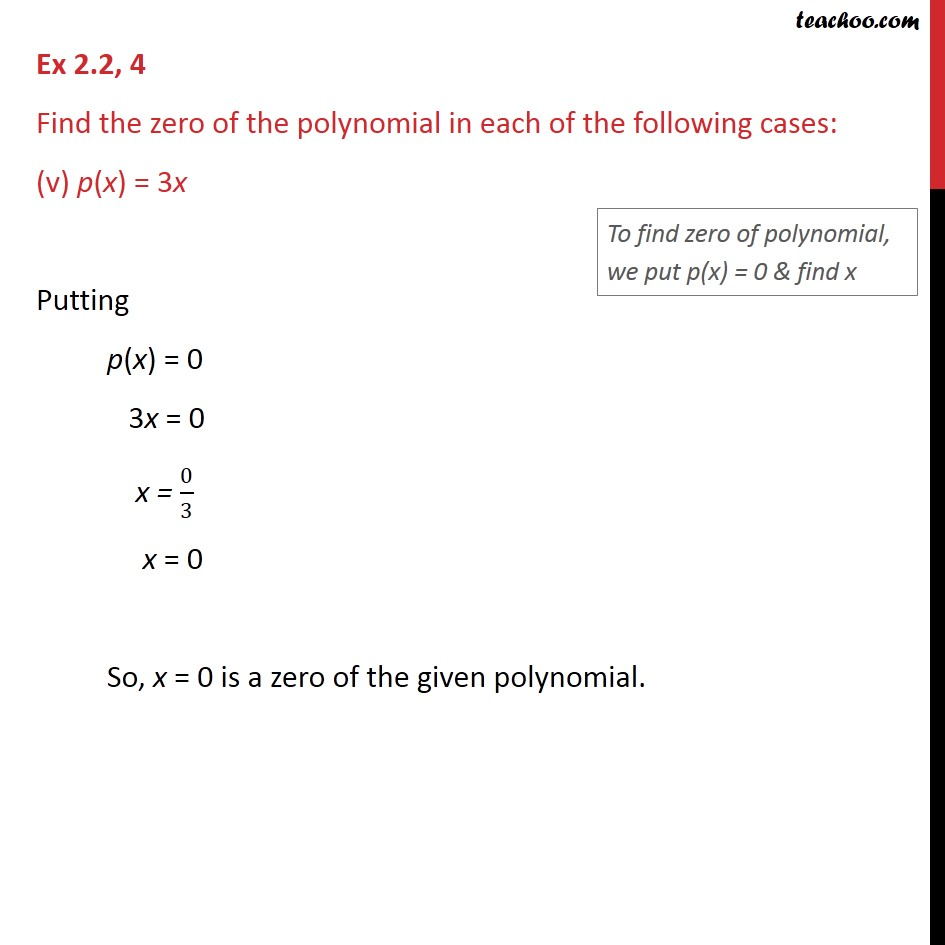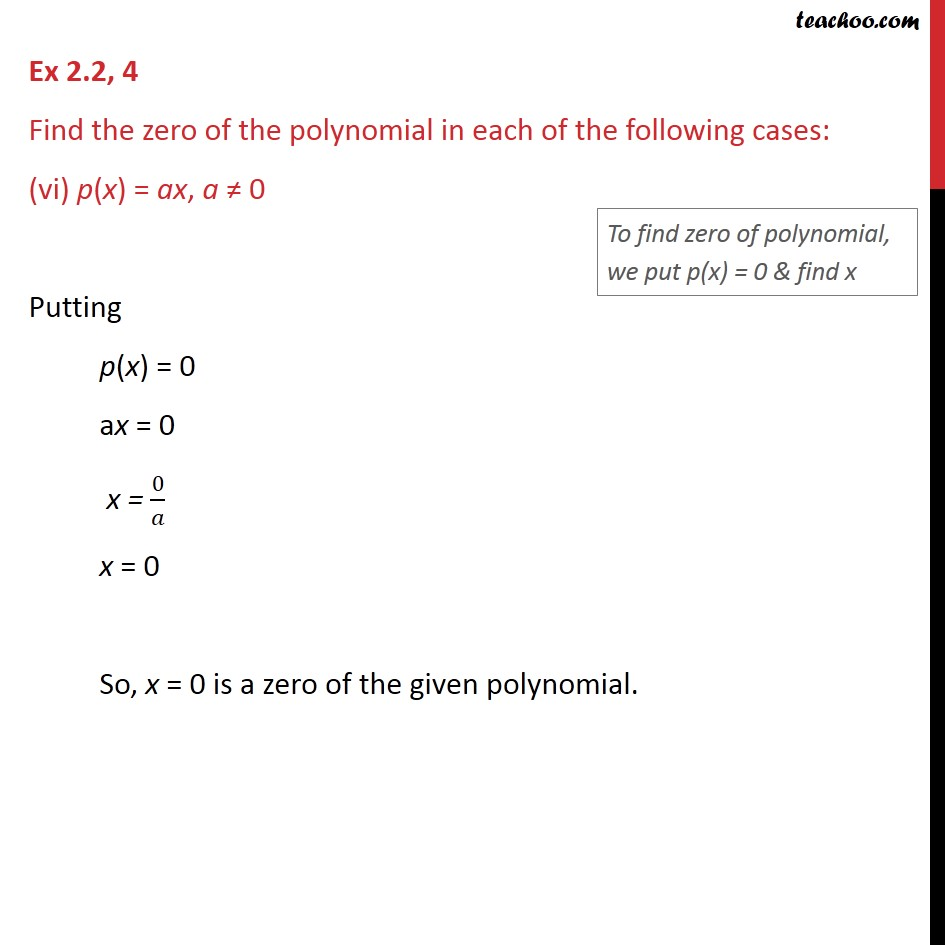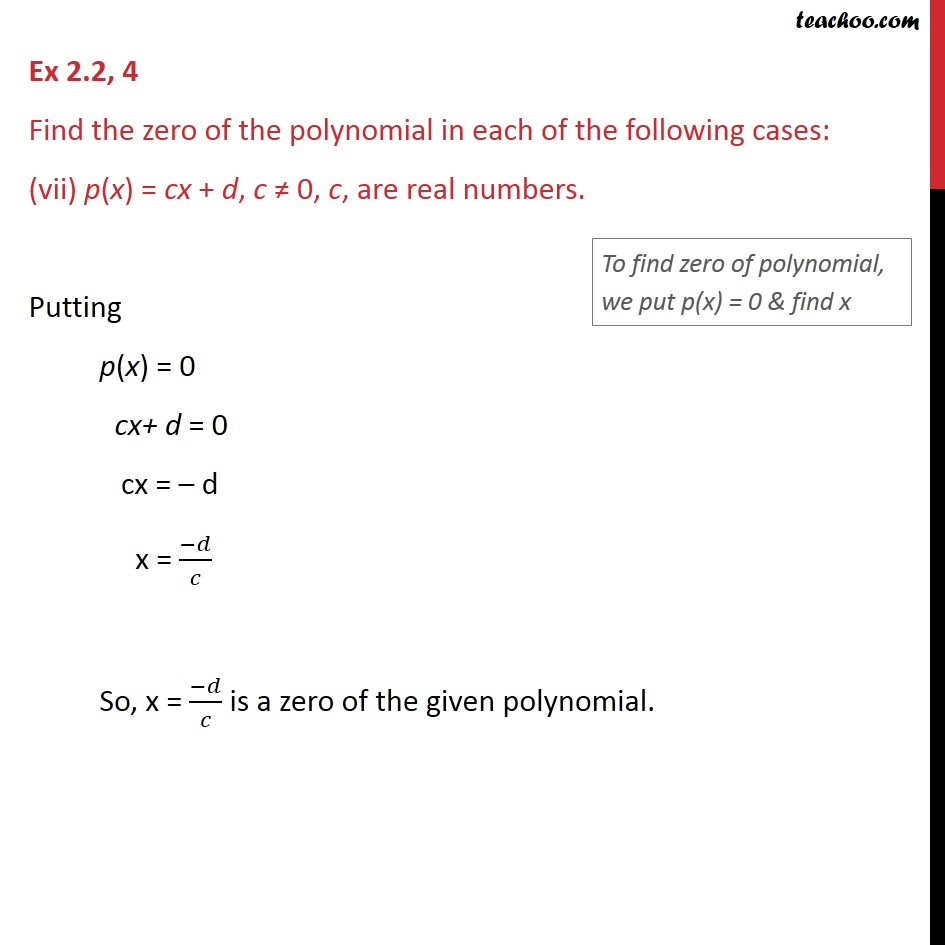1. Chapter 2 Class 9 Polynomials
2. Serial order wise
3. Ex 2.2

Transcript

Ex 2.2, 4 Find the zero of the polynomial in each of the following cases: (i) p(x) = x + 5 Putting p(x) = 0 x + 5 = 0 x = − 5 So, x = −5 is a zero of the given polynomial. Ex 2.2, 4 Find the zero of the polynomial in each of the following cases: (ii) p(x) = x − 5 Putting p(x) = 0 x – 5 = 0 x = 5 So, x = 5 is a zero of the given polynomial. Ex 2.2, 4 Find the zero of the polynomial in each of the following cases: (iii) p(x) = 2x + 5 Putting p(x) = 0 2x + 5 = 0 2x = − 5 x = – 5/2 So, x = – 5/2 is a zero of the given polynomial. Ex 2.2, 4 Find the zero of the polynomial in each of the following cases: (iv) p(x) = 3x − 2 Putting p(x) = 0 3x − 2 = 0 3x = 2 x = 2/3 So, x = 2/3 is a zero of the given polynomial. Ex 2.2, 4 Find the zero of the polynomial in each of the following cases: (v) p(x) = 3x Putting p(x) = 0 3x = 0 x = 0/3 x = 0 So, x = 0 is a zero of the given polynomial. Ex 2.2, 4 Find the zero of the polynomial in each of the following cases: (vi) p(x) = ax, a ≠ 0 Putting p(x) = 0 ax = 0 x = 0/𝑎 x = 0 So, x = 0 is a zero of the given polynomial. Ex 2.2, 4 Find the zero of the polynomial in each of the following cases: (vii) p(x) = cx + d, c ≠ 0, c, are real numbers. Putting p(x) = 0 cx+ d = 0 cx = – d x = (−𝑑)/𝑐 So, x = (−𝑑)/𝑐 is a zero of the given polynomial.

Ex 2.2

Chapter 2 Class 9 Polynomials
Serial order wise

About the AuthorDavneet Singh
Davneet Singh is a graduate from Indian Institute of Technology, Kanpur. He has been teaching from the past 10 years. He provides courses for Maths and Science at Teachoo.